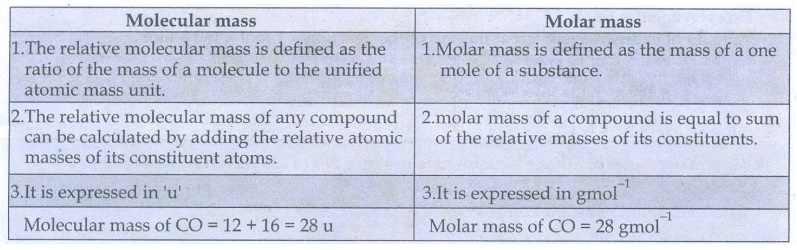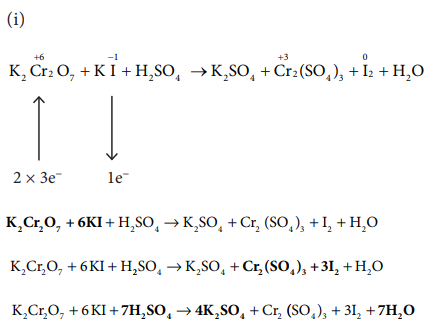Home | | Chemistry 11th std | Brief questions and answers: Basic Concepts of Chemistry and Chemical Calculations

# Brief questions and answers: Basic Concepts of Chemistry and Chemical Calculations

Chemistry : Basic Concepts of Chemistry and Chemical Calculations

Basic Concepts of Chemistry and Chemical Calculations

26) Define relative atomic mass.

The relative atomic mass is defined as the ratio of the average atomic mass factor to the unified atomic mass unit.

Relative atomic mass (Az) :

= Average mass of the atom / Unified atomic mass

27) What do you understand by the term mole.

One mole is the amount of substance of a system, which contains elementary particles as there are atoms in 12g of C-12 isotopes

28) Define equivalent mass.

Gram equivalent mass of an element, compound or ion is the mass that combines or displaces 1.008 g hydrogen (or) 8g oxygen or 35.5g chlorine.

29) What do you understand by the term oxidation number.

Oxidation number is defined as the imaginary charge left on the atom when all other atoms of the compound have been removed in their usual oxidation states.

30) Distinguish between oxidation and reduction.Oxidation

2. Removal of Hydrogen

3. Loss of Electron

4. Increases in Oxidation number

Reduction

1. Removal of Oxygen

3. Gain of Electron

4. Decreases in Oxidation number

31) Calculate the molar mass of the following compounds.

i. urea [CO(NH2)2]

ii. acetone [CH3COCH3]

iii. boric acid [H3BO3]

iv. sulphuric acid [H2SO4]

(i) urea [CO(NH2)2]

N = 2 × 14 = 28

H = 4 × 1 = 4

C = 1 × 12 = 12

O = 1 × 16 = 16

====

60

=====

Molar mass of urea : 60g mol−1

(ii) acetone [CH3COCH3]

C = 3 × 12 = 36

H = 6 × 1 = 6

O = 1 × 16 = 16

=====

58

=====

Molar mass of acetone = 58g mol−1

(iii) boric acid [H3BO3]

B = 1 × 11 = 11

H = 3 × 1 = 3

O = 3 × 16 = 48

=========

62

=========

Molar mass of Boricacid : 62g mol−1

(iv) sulphuric acid [H2SO4]

S = 1 × 32 = 32

H = 2 × 1 = 2

O = 4 × 16 = 64

==========

98

==========

Molar mass of H2SO4 = 98g mol−1

32) The density of carbon dioxide is equal to 1.965 kgm-3 at 273 K and 1 atm pressure. Calculate the molar mass of CO2.

Given:

The density of CO2 at 273K and

1 atm pressure = 1.965 kgm−3

Molar mass of CO2 = ?

At 273K and 1 atm pressure,

1 mole of CO2 occupies a volume of 22.4l

Mass of 1 mole of CO2 = ( l.965K.g /1m3 ) × 22.4L

= [ 1.965 × 103g × 22.4 × 10−3m3 ] / 1m3 = 44.01 g

Molar mass of CO2 = 44.01 g mol−1

33) Which contains the greatest number of moles of oxygen atoms

i) 1 mol of ethanol

ii) 1 mol of formic acid

iii) 1 mol of H2O34) Calculate the average atomic mass of naturally occurring magnesium using the following dataSolution:

Average atomic mass =

[ (78.99 × 23.99) + (10 × 24.99) + (11.01 × 25.98) ] / 100

= 2430.9 / 100 = 24.31u

35) In a reaction x + y + z2  xyz2 identify the Limiting reagent if any, in the following reaction mixtures.

(a) 200 atoms of x + 200 atoms of y + 50 molecules of z2

(b) 1mol of x + 1 mol of y+3 mol of z2

(c) 50 atoms of x + 25 atoms of y+50 molecules of z2

(d) 2.5 mol of x +5 mol of y+5 mol of z2

Reaction : x + y + z2  xyz236) Mass of one atom of an element is 6.645 x 10-23 g. How many moles of element are there in 0.320 kg.

Solution:

Given : mass of one atom = 6.645× 10−23 g

Mass of 1 mole of atom = 6.645 × 10−23g × 6.022 ×1023 = 40g

mole number of moles of element in 0.320 kg = [1 mole / 40 g ] × 0.320kg = 8 mols

37) What is the difference between molecular mass and molar mass? Calculate the molecular mass and molar mass for carbon monoxide.Molecular mass

l. The relative molecular mass is defined as the ratio of the mass of a molecule to the unified atomic mass unit.

2. The relative molecular mass of any compound can be calculated by adding the relative atomic masses of its constituent atoms.

3. It is expressed in 'u'

Molecular mass of CO = 12 + 16 = 28 u

Molar mass

1. Molar mass is defined as the mass of a one mole of a substance.

2. molar mass of a compound is equal to sum of the relative masses of its constituents.

3. It is expressed in gmol−1

Molar mass of CO = 28 gmol−1

38) What is the empirical formula of the following ?

i) Fructose (C6H12O6) found in honey

ii) Caffeine (C8H10N4O2) a substance found in tea and coffee.Fructose

Empirical formula : C6H12O6

Molecular formula : CH2O

Caffeine

Empirical formula : C8H10N4O2

Molecular formula : C4H5N2

39) The reaction between aluminium and ferric oxide can generate temperatures up to 3273 K and is used in welding metals. (Atomic mass of AC = 27 u Atomic mass of 0 = 16 u)

2Al + Fe2O3  Al2O3 +2Fe; If, in this process, 324 g of aluminium is allowed to react with 1.12 kg of ferric oxide.

i) Calculate the mass of Al2O3 formed.

ii) How much of the excess reagent is left at the end of the reaction?40) How many moles of ethane is required to produce 44 g of CO2 (g) after combustion.

Solution:

Balanced equation for the combustion of ethane

C2H6+ 7/2O2 → 2CO2+ 3H2O

To produce 2 moles of CO2, 1 moles of ethane is required

To produce 1 mole 44 g of CO2 required

number of moles of ethane = [ 1 mol ethane / 2 mole CO2 ] × 1 mol CO2

= ½ mole of ethane

= 0.5 mole of ethane

41) Hydrogen peroxide is an oxidising agent. It oxidises ferrous ion to ferric ion and reduced itself to water. Write a balanced equation.1 H2O2 + 2Fe2+  Fe3+ + H2O

H2O2 + 2Fe2+ + 2H+  2Fe3+ + 2H2O

42) Calculate the empirical and molecular formula of a compound containing 76.6% carbon, 6.38 % hydrogen and rest oxygen its vapour density is 47.43) A Compound on analysis gave Na = 14.31% S = 9.97% H= 6.22% and O= 69.5% calculate the molecular formula of the compound if all the hydrogen in the compound is present in combination with oxygen as water of crystallization. (molecular mass of the compound is 322).Molecular formula = Na2 S H20 O14

Since all the hydrogen in the compound present as water

Molecular formula is Na2 SO4 . 10H2O

44) Balance the following equations by oxidation number method

i) K2Cr2O7 + KI + H2SO4  K2SO4 + Cr2(SO4)3 +I2+H2O

ii) KMnO4 + Na2SO3  MnO2 + Na2SO4 + KOH

iii) Cu+ HNO3  Cu(NO3)2 + NO2+ H2O

iv) KMnO4+H2C2O4 + H2SO4  K2SO4 + MnSO4 + CO2 + H2O45) Balance the following equations by ion electron method.

i) KMnO4 + SnCl2+HCl  MnCl2 + SnCl4 + H2O + KCl

ii) C2O42_ + Cr2 O72_  Cr3+ + CO2 (in acid medium)

iii) Na2S2O3 + I2  Na2S4O6 + NaI ( in acid medium)

iv) Zn +NO3_  Zn2+ + NO